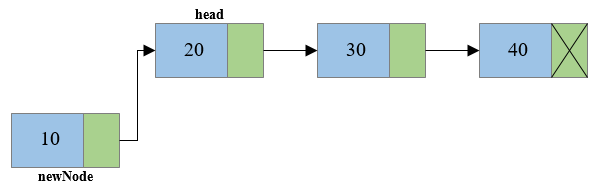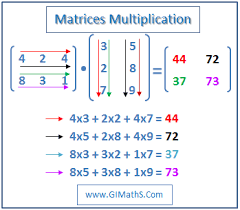## Posts

Showing posts from August, 2019

### How to find and replace a node in Linked ListFor replacing an element in a linked list first we have to find it , for that we will use a function which will do the finding part and give us the node's address (pointer) and then we will replace the value of that node by the value given by the user. Definition of node* find_pos(int) function node* find_pos(int val,int p){ node* cur=first; node* prev=cur; while(cur->val!=val){ prev=cur; cur=cur->next; if(cur==NULL)return NULL; } switch(p){ case 0: return cur; case 1: return cur->next; default:return prev; } } The above function keeps track of the previous node and keep traversing through the linked list until we find the value which is to be replaced, it return the previous node if value of p is -1 or returns current node if p=0 or next one if p=1. Definition of find_replace function void find_replace(int find,int replace){ int c=0; while(node *temp=find_pos(find,0)

### How to Insert ,Delete ( first or last ) nodes in Linked List C / C++Node definition (Structure) : struct node{ int num; node* next; }; The num variable stores the data given by the user and the pointer (next) stores the address of the next element present in the linked list. Insertion of Nodes : 1) Inserting in beginning :  void insert_beg(int value){ node* n=new node; n->num=value; if(first==NULL){ n->next=NULL; first=n; } else{ n->next=first; first=n; } } the above function ,when called creates a new node and stores the value passed to it in num (variable) ,now the function checks if the list is empty and if it is empty then it is simply marked as the first element in the linked list. if the list is not empty then the pointer (next ) is assgned to the first element address and the marker (first) is given to current element. 2) Insertion at end :  void insert_end(int value){ node* n=new node; n->num=value; n->next=

### How to Insert ,Delete nodes in between the Linked ListNode definition (Structure) : struct node{ int num; node* next; }; The num variable stores the data given by the user and the pointer (next) stores the address of the next element present in the linked list. Insertion in between nodes (After a given node ) : void insert_inBetween(){ int cvalue,value; cout<<"Enter the value after which you want to insert the new node"<<endl; cin>>cvalue; cout<<"enter the value of new node: "; cin>>value; cout<<endl; node *n,*prev; n->num=value; if(prev=find(cvalue,0)){ n->next = prev->next; prev->next=n; cout<<"node inserted succesfully"; } else{ cout<<"Node value not found"; } } for performing the insertion after an element given by the user , we need to know the location of the node after which it is to be inserted, for this another function (find()) will be called which will return the address of the node af

### Linked List Implementation ( C ++ ) ProgramA  Linked list  is a linear collection of data elements, whose order is not given by their physical placement in memory. Instead, each element  points  to the next. It is a  data structure  consisting of a collection of  nodes  which together represent a  sequence .  Linked list is an Abstract Data Type ( ADT ). Node definition ( Structure )  struct node{ int num; node* next; }; The num variable stores the data given by the user and the pointer (next) stores the address of the next element present in the linked list. Operations  void insert_beg(int value); void insert_end(int value); void insert_inBetween(); node* find(int cvalue,int p=0); void input_ll(); void display(); void delete_ll(); void delete_node();   Function definitions 1) Inserting in beginning  void insert_beg(int value){ node* n=new node; n->num=value; if(first==NULL){ n->next

### what are Absract Data Types ?An  abstract data type  ( ADT ) is a  mathematical model  for  data types , where a data type is defined by its behavior ( semantics ) from the point of view of a  user  of the data, specifically in terms of possible values, possible operations on data of this type, and the behavior of these operations. This contrasts with  data structures , which are concrete representations of data, and are the point of view of an implementer, not a user. In simple words ADT can be defined as a user defined data type whose operations for read / write are defined by the programmer in the form of functions. Types of Abstract Data Types : 1) A ssociative array ,   map ,   symbol table , or   dictionary   is an   abstract data type   composed of a   collection   of   (key, value) pairs , such that each possible key appears at most once in the collection. Operations associated with this data type allow: the addition of a pair to the collection the removal of a pair from the collection th

### Tower of Hanoi C / C++ / Python ProgramTower of hanoi is a mathematical game or puzzle.  It consists of three rods and a number of disks of different sizes, which can slide onto any rod. The puzzle starts with the disks in a neat stack in ascending order of size on one rod, the smallest at the top, thus making a  conical  shape. The objective of the puzzle is to move the entire stack to another rod, obeying the following simple rules: Only one disk can be moved at a time. Each move consists of taking the upper disk from one of the stacks and placing it on top of another stack or on an empty rod. No larger disk may be placed on top of a smaller disk. With 3 disks, the puzzle can be solved in 7 moves. The minimal number of moves required to solve a Tower of Hanoi puzzle is 2 n  − 1, where  n  is the number of disks. For example consider 3 disks ,it can be solved in 2 3  -1 steps : Steps required to be performed for solving tower of hanoi problem  : The universal way (steps ) to solve this type of pr

### Python and C++ program to implement multiplication of 2d array (Matrix multiplication)Here, in this program we are going to implement matrix multiplication , suppose matrix 1 has dimensions:m*n and matrix 2 has dimensions :p*q for these two matrix to be multiplied we need to have the number of columns in matrix 1(n) equal to the number of rows in matrix 2(p), if the condition is satisfied then the result of multiplication will be a matrix of order m*q in multiplication the elements of the resultant matrix will be the sum of product of corresponding elements of row(of M1) and column(of M2) //first element in 1st row in given example: res+=mat1[i] * mat2[i] where 0<i<n or p //second element in 1st row: res+=mat1[i] * mat2[i] where 0<i<n or p and so on………… Example : Input : 1 2 3 1 4 5 6 2 7 8 9 3 Output : 14 32 50 Input : 4 3 4 2 4 1 1 1 Output : multiplication not possible n!=p Here goes the main execution part where the calculation is been done: for ( int i = 0 ; i < r1 ; i ++) for ( int